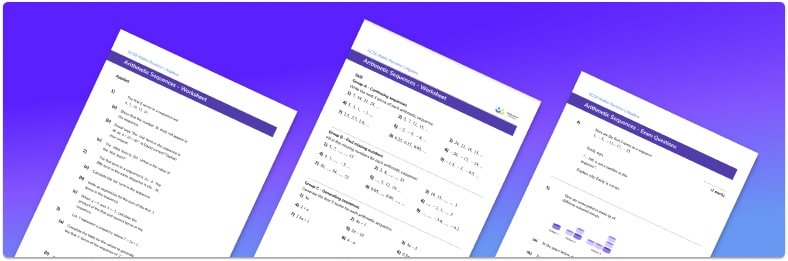# Arithmetic Sequence Worksheet• Section 1 of the arithmetic sequence worksheet contains 27 skills-based arithmetic sequence questions, in 3 groups to support differentiation
• Section 2 contains 3 applied arithmetic sequence questions with a mix of worded problems and deeper problem solving questions
• Section 3 contains 6 foundation and higher level GCSE exam style questions
• Answers and a mark scheme for all questions are provided
• Questions follow variation theory with plenty of opportunities for students to work independently at their own level
• All questions created by fully qualified expert secondary maths teachers
• Suitable for GCSE maths revision for AQA, OCR and Edexcel exam boards

• This field is for validation purposes and should be left unchanged.

You can unsubscribe at any time (each email we send will contain an easy way to unsubscribe). To find out more about how we use your data, see our privacy policy.

### Arithmetic sequence at a glance

Arithmetic sequences are sequences of numbers which increase or decrease by the same amount each time. The numbers may be integers, fractions or decimals and have a common difference which is found by subtracting consecutive terms of an arithmetic sequence. The common difference can be used to find the next term in the sequence or another missing term. It is sometimes known as the term-to-term rule.

The common difference and the first term can be used to find the general term also known as the nth term of the sequence. The nth term allows us to find any term in the sequence by substituting the term number as the value of n, for example we can work out the 10th term by substituting 10 as the value of n.

The nth term can also be used to check if a number is a term in a sequence by setting the number equal to the nth term and solving the equation. If the solution to the linear equation has an integer solution then the term is in the sequence. If the solution is a decimal then the number is not in the sequence.

Looking forward, students can progress with more sequences worksheets to additional algebra worksheets, for example a collecting like terms worksheet  or simultaneous equations worksheet.For more teaching and learning support on Algebra our GCSE maths lessons provide step by step support for all GCSE maths concepts.

## Do you have KS4 students who need more focused attention to succeed at GCSE?There will be students in your class who require individual attention to help them succeed in their maths GCSEs. In a class of 30, it’s not always easy to provide.

Help your students feel confident with exam-style questions and the strategies they’ll need to answer them correctly with our dedicated GCSE maths revision programme.

Lessons are selected to provide support where each student needs it most, and specially-trained GCSE maths tutors adapt the pitch and pace of each lesson. This ensures a personalised revision programme that raises grades and boosts confidence.

Find out more